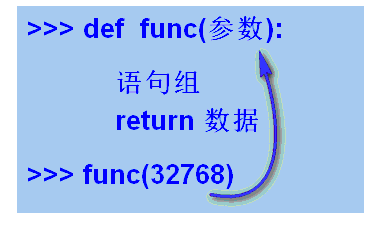# python有参函数教程## 有参函数(Parametric Function)```>>> def add(x,y):      #  定义函数，有两个参数
z = x + y            # 把参数的值加起来赋值给z
return z             # 返回z

>>> s = add(32,76)     # 调用函数，返回结果用s进行引用
>>> s
108
```

```>>> def do_stuff(first,second,third):
string = "小明" + first + "后" + second  +"再" + third
return string

>>> do_stuff("吃饭","刷牙","起床")
'小明吃饭后刷牙再起床'
>>> do_stuff("起床","刷牙","吃饭")
'小明起床后刷牙再吃饭'
>>>
```

## 形参与实参(Formal and Actual parameter)

```>>> def zsum(number):               #  定义函数，有形式参数为number
s = 0
for n in range(1,number+1):
s = s + n
return s

>>> zsum(10)                  # 调用函数，10 是实际参数
55
>>>
```

```>>> def times(number):
number = number * number
return number

>>> times(8)
64
>>> times(16)
256
>>>
```

scratch